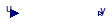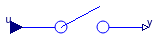## Buildings.Controls.OBC.CDL.Discrete

Package with discrete blocks

### Information

This package contains discrete control blocks with fixed sample period. Every component of this package is structured in the following way:

1. A component has continuous Real input and output signals.
2. The input signals are sampled by the given sample period defined via parameter `samplePeriod`. The first sample instant is defined by the parameter `startTime`.
3. The output signals are computed from the sampled input signals.

### Package Content

Name DescriptionDayType Block that outputs a signal that indicates week-day or week-endFirstOrderHold First order hold of a sampled-data systemMovingMean Discrete moving mean of a sampled input signalSampler Ideal sampler of a continuous signalTriggeredMax Output the maximum, absolute value of a continuous signal at trigger instantsTriggeredSampler Triggered sampling of continuous signalsUnitDelay Output the input signal with a unit delayZeroOrderHold Output the input signal with a zero order holdExamples Collection of models that illustrate model use and test models

##Buildings.Controls.OBC.CDL.Discrete.DayType

Block that outputs a signal that indicates week-day or week-end### Information

This block outputs a signal that indicates the type of the day. It can for example be used to generate a signal that indicates whether the current time is a work day or a non-working day. The output signal is of type Buildings.Controls.OBC.CDL.Types.Day.

The parameter `nout` determines how many days should be sent to the output. For applications in which only the current day is of interest, set `nout=1`. For applications in which the load is predicted for the next 24 hours, set `nout=2` in order to output the type of day for today and for tomorrow.

The transition from one day type to another always happens when the simulation time is a multiple of 1 day. Hence, if the simulation starts for example at t=-3600 seconds, then the first transition to another day will be at t=0.

### Parameters

TypeNameDefaultDescription
Integernout2Number of days to output. Set to two for one day predictions
Daydays[:]{Buildings.Controls.OBC.CDL....Array where each element is a day indicator
IntegeriStart1Index of element in days at simulation start

### Connectors

TypeNameDescription
output DayTypeOutputy[nout]Type of the day for the current and the next (nout-1) days

### Modelica definition

model DayType "Block that outputs a signal that indicates week-day or week-end" parameter Integer nout = 2 "Number of days to output. Set to two for one day predictions"; parameter Buildings.Controls.OBC.CDL.Types.Day[:] days={ Buildings.Controls.OBC.CDL.Types.Day.WorkingDay, Buildings.Controls.OBC.CDL.Types.Day.WorkingDay, Buildings.Controls.OBC.CDL.Types.Day.WorkingDay, Buildings.Controls.OBC.CDL.Types.Day.WorkingDay, Buildings.Controls.OBC.CDL.Types.Day.WorkingDay, Buildings.Controls.OBC.CDL.Types.Day.NonWorkingDay, Buildings.Controls.OBC.CDL.Types.Day.NonWorkingDay} "Array where each element is a day indicator"; parameter Integer iStart(min=1, max=size(days, 1)) = 1 "Index of element in days at simulation start"; Interfaces.DayTypeOutput y[nout] "Type of the day for the current and the next (nout-1) days"; protected parameter Modelica.SIunits.Time samplePeriod=86400 "Sample period of component"; output Integer iDay(min=1, max=size(days, 1)) "Pointer to days that determines what day type is sent to the output"; parameter Modelica.SIunits.Time firstSample(fixed=false) "Time when the sampling starts"; output Boolean sampleTrigger "True, if sample time instant"; output Boolean skipIDayIncrement "If true, don't increment iDay in first sample"; initial equation iDay = iStart; firstSample = ceil(time/86400)*86400; // skipIDayIncrement is true if the simulation starts at midnight. skipIDayIncrement = abs(firstSample-time) < 1E-8; equation for i in 1:nout loop y[i] = days[ mod(iDay+i-2, size(days, 1))+1]; end for; sampleTrigger = sample(firstSample, samplePeriod); when sampleTrigger then skipIDayIncrement = false; if pre(skipIDayIncrement) then iDay = pre(iDay); else iDay = mod(pre(iDay), size(days, 1))+1; end if; end when; end DayType;

##Buildings.Controls.OBC.CDL.Discrete.FirstOrderHold

First order hold of a sampled-data system### Information

Block that outputs the extrapolation through the values of the last two sampled input signals.

### Parameters

TypeNameDefaultDescription
TimesamplePeriodsamplePeriod( ...Sample period of component [s]

### Connectors

TypeNameDescription
input RealInputuContinuous input signal
output RealOutputyContinuous output signal

### Modelica definition

block FirstOrderHold "First order hold of a sampled-data system" parameter Modelica.SIunits.Time samplePeriod(min=1E-3) "Sample period of component"; Interfaces.RealInput u "Continuous input signal"; Interfaces.RealOutput y "Continuous output signal"; protected parameter Modelica.SIunits.Time t0(fixed=false) "First sample time instant"; output Boolean sampleTrigger "True, if sample time instant"; output Boolean firstTrigger(start=false, fixed=true) "Rising edge signals first sample instant"; Modelica.SIunits.Time tSample "Time of sample"; Real uSample "Value of sample"; Real pre_uSample "Value of previous sample"; Real c "Slope"; initial equation t0 = time; pre(tSample) = time; pre(uSample) = u; pre(pre_uSample) = u; pre(c) = 0.0; equation // Declarations that are used for all discrete blocks sampleTrigger = sample(t0, samplePeriod); when sampleTrigger then firstTrigger = time <= t0 + samplePeriod/2; end when; // Declarations specific to this type of discrete block when sampleTrigger then tSample = time; uSample = u; pre_uSample = pre(uSample); c = if firstTrigger then 0 else (uSample - pre_uSample)/samplePeriod; end when; /* Use pre_uSample and pre(c) to break potential algebraic loops by an infinitesimal delay if both the continuous and the discrete part have direct feedthrough. */ y = pre_uSample + pre(c)*(time - tSample); end FirstOrderHold;

##Buildings.Controls.OBC.CDL.Discrete.MovingMean

Discrete moving mean of a sampled input signal### Information

Block that outputs the sampled moving mean value of an input signal. At each sampling instant, the block outputs the average value of the past n samples including the current sample.

At the first sample, the block outputs the first sampled input. At the next sample, it outputs the average of the past two samples, then the past three samples and so on up to n samples.

### Parameters

TypeNameDefaultDescription
Integernn( min=2)Number of samples over which the input is averaged
TimesamplePeriodsamplePeriod( ...Sampling period of component [s]

### Connectors

TypeNameDescription
input RealInputuContinuous input signal
output RealOutputyDiscrete averaged signal

### Modelica definition

block MovingMean "Discrete moving mean of a sampled input signal" parameter Integer n(min=2) "Number of samples over which the input is averaged"; parameter Modelica.SIunits.Time samplePeriod(min=1E-3) "Sampling period of component"; Interfaces.RealInput u "Continuous input signal"; Interfaces.RealOutput y "Discrete averaged signal"; protected parameter Modelica.SIunits.Time t0(fixed=false) "First sample time instant"; Boolean sampleTrigger "Trigger samples at each sampling instant"; Integer iSample(start=0, fixed=true) "Sample numbering in the simulation"; Integer counter(start=0, fixed=true) "Number of samples used for averaging calculation"; Integer index(start=0, fixed=true) "Index of the vector ySample"; discrete Real ySample[n]( start=vector(zeros(n,1)), each fixed=true) "Vector of samples to be averaged"; initial equation t0 = time; y = u; equation sampleTrigger = sample(t0, samplePeriod); algorithm when sampleTrigger then index := mod(iSample, n) + 1; ySample[index] := u; counter := if counter == n then n else counter + 1; y := sum(ySample)/counter; iSample := iSample + 1; end when; end MovingMean;

##Buildings.Controls.OBC.CDL.Discrete.Sampler

Ideal sampler of a continuous signal### Information

Block that outputs the input signal, sampled at a sampling rate defined via parameter `samplePeriod`.

### Parameters

TypeNameDefaultDescription
TimesamplePeriodsamplePeriod( ...Sample period of component [s]

### Connectors

TypeNameDescription
input RealInputuContinuous input signal
output RealOutputyContinuous output signal

### Modelica definition

block Sampler "Ideal sampler of a continuous signal" parameter Modelica.SIunits.Time samplePeriod(min=1E-3) "Sample period of component"; Interfaces.RealInput u "Continuous input signal"; Interfaces.RealOutput y "Continuous output signal"; protected parameter Modelica.SIunits.Time t0(fixed=false) "First sample time instant"; output Boolean sampleTrigger "True, if sample time instant"; output Boolean firstTrigger(start=false, fixed=true) "Rising edge signals first sample instant"; initial equation t0 = time; equation // Declarations that are used for all discrete blocks sampleTrigger = sample(t0, samplePeriod); when sampleTrigger then firstTrigger = time <= t0 + samplePeriod/2; end when; // Declarations specific to this type of discrete block when {sampleTrigger, initial()} then y = u; end when; end Sampler;

##Buildings.Controls.OBC.CDL.Discrete.TriggeredMax

Output the maximum, absolute value of a continuous signal at trigger instants### Information

Block that outputs the input signal whenever the trigger input signal is rising (i.e., trigger changes to `true`). The maximum, absolute value of the input signal at the sampling point is provided as the output signal.

### Connectors

TypeNameDescription
input RealInputuConnector with a Real input signal
output RealOutputyConnector with a Real output signal
input BooleanInputtriggerConnector for trigger

### Modelica definition

block TriggeredMax "Output the maximum, absolute value of a continuous signal at trigger instants" Interfaces.RealInput u "Connector with a Real input signal"; Interfaces.RealOutput y "Connector with a Real output signal"; Interfaces.BooleanInput trigger "Connector for trigger"; initial equation y = u; equation when trigger then y = max(pre(y), abs(u)); end when; end TriggeredMax;

##Buildings.Controls.OBC.CDL.Discrete.TriggeredSampler

Triggered sampling of continuous signals### Information

Samples the continuous input signal whenever the trigger input signal is rising (i.e., trigger changes from `false` to `true`) and provides the sampled input signal as output. Before the first sampling, the output signal is equal to the initial value defined via parameter `y_start`.

### Parameters

TypeNameDefaultDescription
Realy_start0Initial value of output signal

### Connectors

TypeNameDescription
input RealInputuConnector with a Real input signal
output RealOutputyConnector with a Real output signal
input BooleanInputtriggerSignal that triggers the sampler

### Modelica definition

block TriggeredSampler "Triggered sampling of continuous signals" parameter Real y_start=0 "Initial value of output signal"; Interfaces.RealInput u "Connector with a Real input signal"; Interfaces.RealOutput y "Connector with a Real output signal"; Interfaces.BooleanInput trigger "Signal that triggers the sampler"; initial equation y = y_start; equation when trigger then y = u; end when; end TriggeredSampler;

##Buildings.Controls.OBC.CDL.Discrete.UnitDelay

Output the input signal with a unit delay### Information

Block that outputs the input signal with a unit delay:

```          1
y = --- * u
z
```

that is, the output signal `y` is the input signal `u` of the previous sample instant. Before the second sample instant, the output `y` is identical to parameter `y_start`.

### Parameters

TypeNameDefaultDescription
TimesamplePeriodsamplePeriod( ...Sample period of component [s]
Realy_start0Initial value of output signal

### Connectors

TypeNameDescription
input RealInputuContinuous input signal
output RealOutputyContinuous output signal

### Modelica definition

block UnitDelay "Output the input signal with a unit delay" parameter Modelica.SIunits.Time samplePeriod(min=1E-3) "Sample period of component"; parameter Real y_start=0 "Initial value of output signal"; Interfaces.RealInput u "Continuous input signal"; Interfaces.RealOutput y "Continuous output signal"; protected parameter Modelica.SIunits.Time t0(fixed=false) "First sample time instant"; output Boolean sampleTrigger "True, if sample time instant"; output Boolean firstTrigger(start=false, fixed=true) "Rising edge signals first sample instant"; initial equation t0 = time; y = y_start; equation // Declarations that are used for all discrete blocks sampleTrigger = sample(t0, samplePeriod); when sampleTrigger then firstTrigger = time <= t0 + samplePeriod/2; end when; // Declarations specific to this type of discrete block when sampleTrigger then y = pre(u); end when; end UnitDelay;

##Buildings.Controls.OBC.CDL.Discrete.ZeroOrderHold

Output the input signal with a zero order hold### Information

Block that outputs the sampled input signal at sample time instants. The output signal is held at the value of the last sample instant during the sample points. At initial time, the block feeds the input directly to the output.

### Parameters

TypeNameDefaultDescription
TimesamplePeriodsamplePeriod( ...Sample period of component [s]

### Connectors

TypeNameDescription
input RealInputuContinuous input signal
output RealOutputyContinuous output signal

### Modelica definition

block ZeroOrderHold "Output the input signal with a zero order hold" parameter Modelica.SIunits.Time samplePeriod(min=1E-3) "Sample period of component"; Interfaces.RealInput u "Continuous input signal"; Interfaces.RealOutput y "Continuous output signal"; protected parameter Modelica.SIunits.Time t0(fixed=false) "First sample time instant"; output Real ySample(fixed=true, start=0) "Sampled value of input"; output Boolean sampleTrigger "True, if sample time instant"; output Boolean firstTrigger(start=false, fixed=true) "Rising edge signals first sample instant"; initial equation t0 = time; equation // Declarations that are used for all discrete blocks sampleTrigger = sample(t0, samplePeriod); when sampleTrigger then firstTrigger = time <= t0 + samplePeriod/2; end when; // Declarations specific to this type of discrete block when {sampleTrigger, initial()} then ySample = u; end when; /* Define y=ySample with an infinitesimal delay to break potential algebraic loops if both the continuous and the discrete part have direct feedthrough */ y = pre(ySample); end ZeroOrderHold;